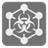Network Attack
Elementary+
English FR JA RUNicola regularly inspects the local networks for security issues. He uses a smart and aggressive program which takes control of computers on the network. This program attacks all connected computers simultaneously, then uses the captured computers for further attacks. Nicola started the virus program in the first computer and took note of the time it took to completely capture the network. We can help him improve his process by modeling and improving his inspections.

We are given information about the connections in the network and the security level for each computer. Security level is the time (in minutes) that is required for the virus to capture a machine. Capture time is not related to the number of infected computers attacking the machine. Infection start from the 0th computer (which is already infected). Connections in the network are undirected. Security levels are not equal to zero (except infected).

Information about a network is represented as a matrix NxN size, where N is a number of computers. If ith computer connected with jth computer, then matrix[i][j] == matrix[j][i] == 1, else 0. Security levels are placed in the main matrix diagonal, so matrix[i][i] is the security level for the ith computer.You should calculate how much time is required to capture the whole network in minutes.

Input: Network information as a list of lists with integers.

Output: The total time of taken to capture the network as an integer.

Example:

```capture([[0, 1, 0, 1, 0, 1],
[1, 8, 1, 0, 0, 0],
[0, 1, 2, 0, 0, 1],
[1, 0, 0, 1, 1, 0],
[0, 0, 0, 1, 3, 1],
[1, 0, 1, 0, 1, 2]]) == 8
capture([[0, 1, 0, 1, 0, 1],
[1, 1, 1, 0, 0, 0],
[0, 1, 2, 0, 0, 1],
[1, 0, 0, 1, 1, 0],
[0, 0, 0, 1, 3, 1],
[1, 0, 1, 0, 1, 2]]) == 4
capture([[0, 1, 1],
[1, 9, 1],
[1, 1, 9]]) == 9
```

How it is used: This concept shows how to model and examine various network configurations. The idea does not only apply to computer networks however, it can also be a model for the spread of disease or dissemination of rumors.

Precondition:

```3 ≤ len(matrix) ≤ 10
matrix == 0
all(len(row) == len(matrix) for row in matrix)
all(matrix[i][j] == matrix[j][i] for i in range(len(matrix)) for j in range(len(matrix)))
all(0 < matrix[i][i] < 10 for i in range(1, len(matrix)))
all(0 ≤ matrix[i][j] ≤ 1 for i in range(len(matrix)) for j in range(len(matrix)) if i != j)
```